# An Example Of A Trading Strategy Coded Using Quantmod Package In R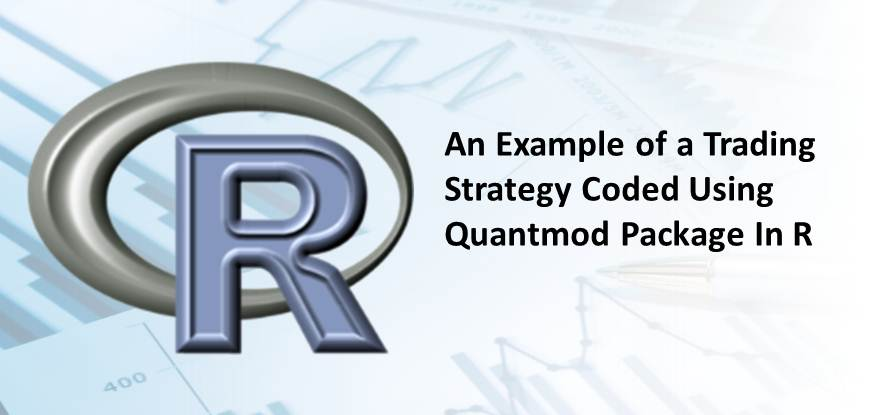In this post, we will back-test our trading strategy in R. Back-testing of a trading strategy can be implemented in four stages.

### Getting the historical data

The quantmod package has made it really easy to pull historical data from Yahoo Finance. The one line code below fetches NSE ( Nifty) data.
`getSymbols("^NSEI")`
Quantmod provides various features to visualize data. The command below creates chart for the NSE data.
`chartSeries(NSEI, TA=NULL)`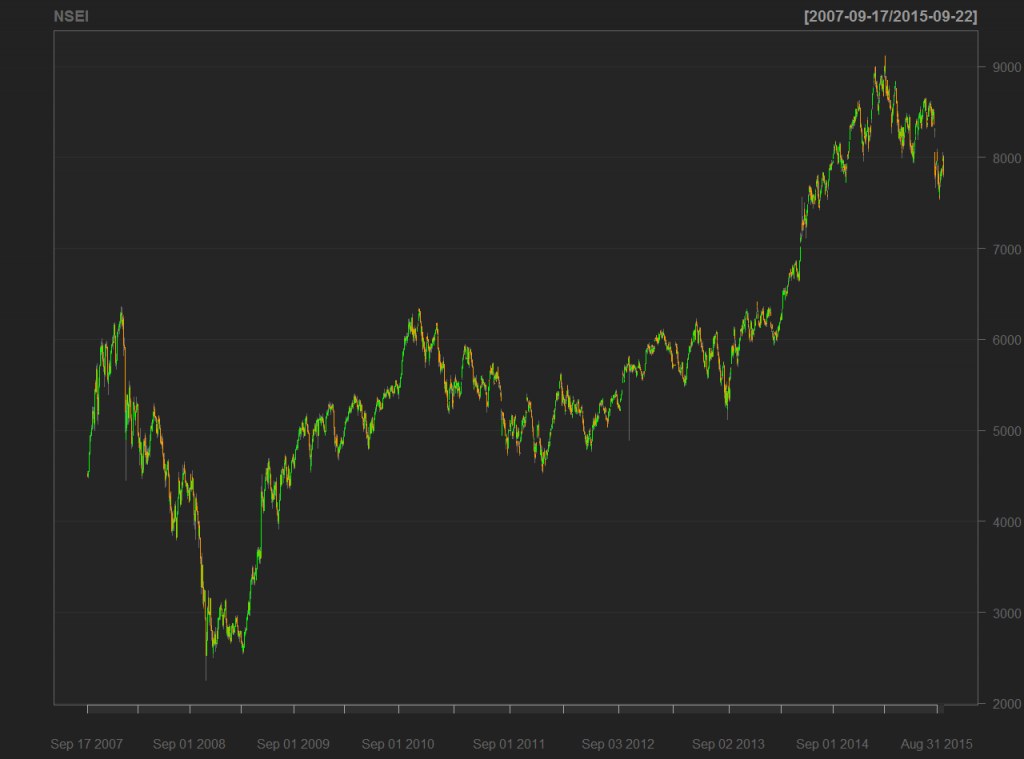TA=”Null” indicates not to use any technical indicator. We will see shortly application of a technical indicator on a chart.

### Formulate the trading strategy and specify the rules

Next step is to pick a trading strategy. We will choose MACD (Moving Average Convergence Divergence) for this example. In a moving average crossovers strategy two averages are computed, a slow moving average and a fast moving average. The difference between the fast moving average and the slow moving average is called MACD line.  A third average called signal line; a 9 day exponential moving average of MACD signal, is also computed. If the MACD line crosses above the signal line then it is a bullish sign and we go long. If the MACD line crosses below the signal line then it is a bearish sign and we go short. We choose the closing price of NSE data to calculate the averages. Following command fulfils this task.
`data=NSEI[,4]`
The command below computes the MACD for the closing price.
`macd = MACD(data, nFast=12, nSlow=26,nSig=9,maType=SMA, percent = FALSE)`
One can choose varying parameters for fast, slow and signal averages depending upon the trading requirements. Here we stick to the standard parameters. MACD is the function in quantmod that calculates the moving average convergence divergence, data is the closing price for NSE, nFast is the fast moving average, nSlow is the slow moving average, maType =SMA indicates we have chosen simple moving average, percent =FALSE implies we are calculating the difference between fast moving average and slow moving average. Setting it TRUE would return the percentage difference between the fast moving average and slow moving average.

The following command plots the chart for the closing price of NSE along with the MACD parameters.

`chartSeries(NSEI, TA="addMACD()")`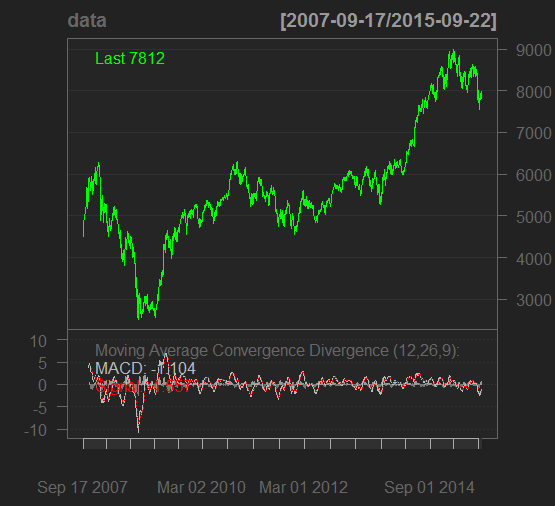As discussed before we define our trading signal as follows:-

• If the MACD signal crossed above the signal line we go long on NSE
• If the MACD signal crossed below the signal line we go short on NSE
Following command generates the trading signal accordingly. We use the lag operator to eliminate look ahead bias.

signal = Lag(ifelse(macd\$macd < macd\$signal, -1, 1))

### Execute the strategy on the historical data

We will apply this strategy on the historical data of NSE from 2007-09-17 to 2015-09-22. The trading signal is applied to the closing price to obtain the returns of our strategy.
`returns = ROC(data)*signal`
The ROC function provides the percentage difference between the two closing prices. We can choose the duration for which we want to see the returns. The following command chooses the returns between 2008-06-02 and 2015-09-22.

Cumulative returns can be calculated and plotted using the following commands:-

```portfolio = exp(cumsum(returns))

plot(portfolio)```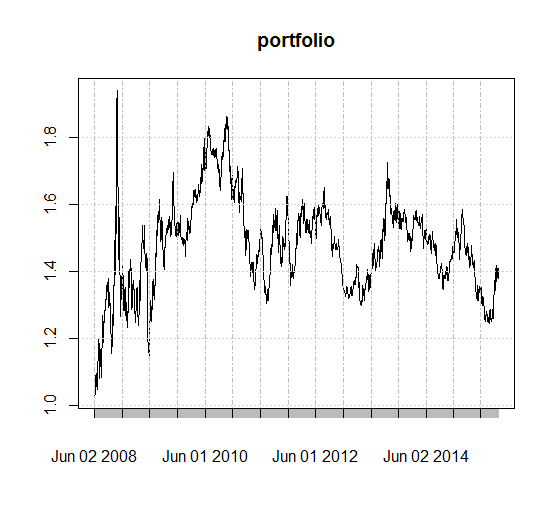### Evaluate performance metrics

The 4th step of back-testing is evaluating performance metrics. The performance analytics package in R provides a consolidated platform to observe performance related parameters. Various metrics like draw-downs, downside risk can be observed in R.

Following command provides a summary of the above-mentioned parameters and much more!

```table.Drawdowns(ret, top=10)

table.DownsideRisk(ret)

charts.PerformanceSummary(ret)```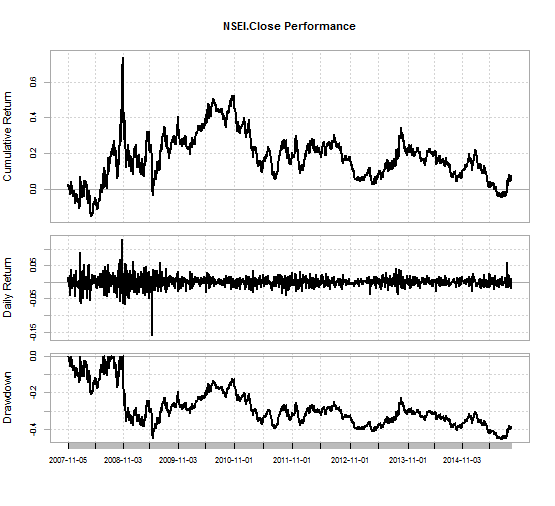Here is the succinct version of the code.

```require(quantmod)
require(PerformanceAnalytics)
getSymbols('^NSEI')
chartSeries(NSEI, TA=NULL)
data=NSEI[,4]
macd = MACD(data, nFast=12, nSlow=26,nSig=9,maType=SMA,percent = FALSE)
signal = Lag(ifelse(macd\$macd < macd\$signal, -1, 1))
returns = ROC(data)*signal
returns = returns['2008-06-02/2015-09-22']
portfolio = exp(cumsum(returns))
plot(portfolio)
table.Drawdowns(returns, top=10)
table.DownsideRisk(returns)
charts.PerformanceSummary(returns)```

### Next Step

In this post we illustrated a very simple strategy and saw how you can break down a premise into the reaction to events. Just to make it more simple we have added an example of how this trading strategy is coded in C++. Click here to access now. In addition to this, you can check our blog for articles on different quantitative trading strategies.

Update

We have noticed that some users are facing challenges while downloading the market data from Yahoo and Google Finance platforms. In case you are looking for an alternative source for market data, you can use Quandl for the same.